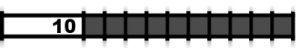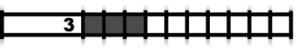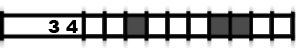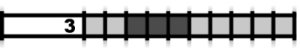Hanjie puzzle, picross game rules

In order to solve picross puzzles, you need to know the game rules first. Once you understand the rules, hours of puzzles are waiting for you! Be careful! Playing hanjie might become an addiction!

Goal of the game

The goal in hanjie is to blacken the squares of the puzzle in order to reveal an image, a drawing. The numbers on the left side and above the puzzle give you a hint to help you deduce which square has to be blackened.

Which square to blacken?

The numbers on the left side of the puzzle indicate how many squares you need to blacken on the matching line.
The numbers above the puzzle indicate how many squares you need to blacken on the matching column.

What are these numbers?

A 5 in front of a line (that means on the left side of the puzzle) indicates that you have to blacken five squares one behind the other on this line.The sequence 3 2 means that there are at least one empty square between three black squares and two black squares sequences.Easy black squares

There are a few tricks to know to help you solve quickly a picross puzzle.
For instance if the picross puzzle is a 10 by 10 square, a column (or line) that indicates 10 means that all the squares on that column (or line) have to be blackened.If the first square of a line (or a column) is black and if the first number of this line is 3, then you can blacken the two following squares because the 3 necessarily matches the three fisrt squares of the line. This trick also applies to the end of a line or column.One other trick: if a line (or column) is 10 squares, and if only 7 of those have to be blackened, then you can blacken the four central squares. In fact, those squares will be blackened whatever is the solution for this line (or column). This trick works as soon as a line or a column only has one number and if this number is higher than half the squares of the line (or column).One last trick, a harder one. Stay focused! Let's imagine that a line is 10 squares and that the numbers are 3 4. We know that there is at least one empty square between the two series. Let's think to those series as a whole, which makes it 8 squares to blacken: 3 black squares + 1 empty square (hypothesis) + 4 black squares. That means that 2 will remain empty. The line must start with 0, 1 or 2 empty squares. Also, this line must end with 0, 1 or 2 empty squares. Thus we can blacken the third, the seventh and the eighth squares because whatever is the solution those squares will end black.
Try to imagine the different solutions on the following picture and you will see by yourself!Squares that remain empty

You can check the squares that will remain empty, this helps you solving the picross puzzle.
For instance if a line contains three black squares and that you already have blackened them, you can check all the remaining squares of this linde. In the end they will represent the white parts on the picture.Going "hypothesis"

There might be a moment when you will be stuck and you won't know which case to blacken. At this moment you can go into the "hypothesis" mode. This mode changes the color of the squares you will temporarily blacken or check and will help you see any mistake.
The "hypothesis" mode allows you to make some progress in the solving of the picross puzzle thanks to a hypothesis, but you can also go back and cancel all of the "hypothesis" squares.